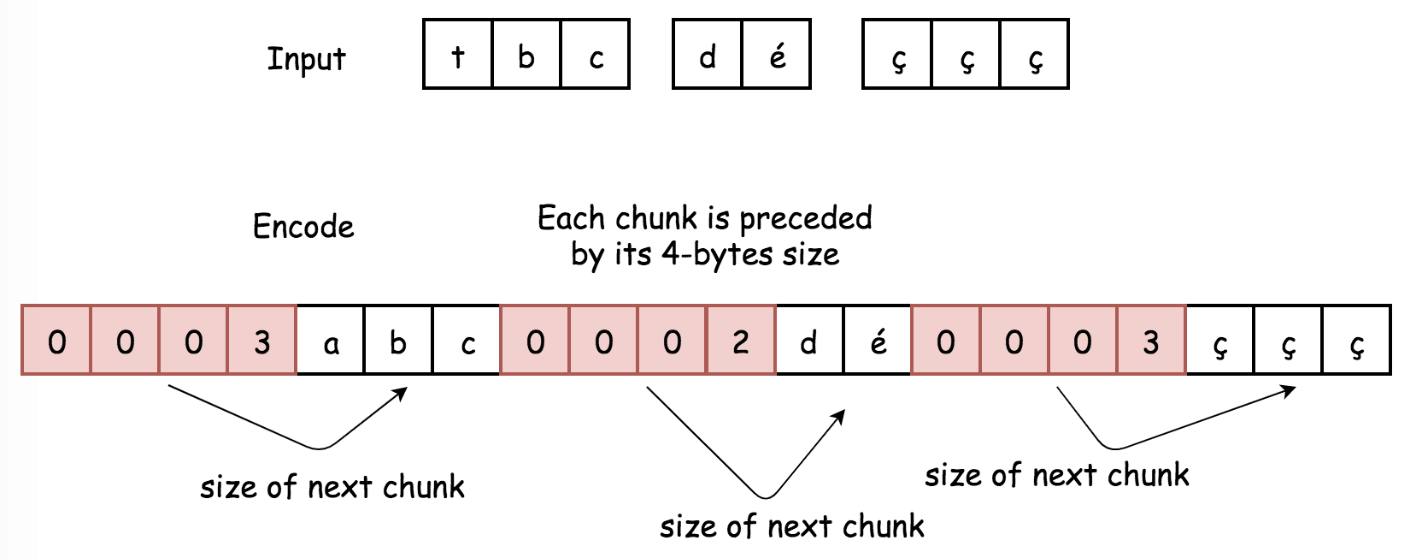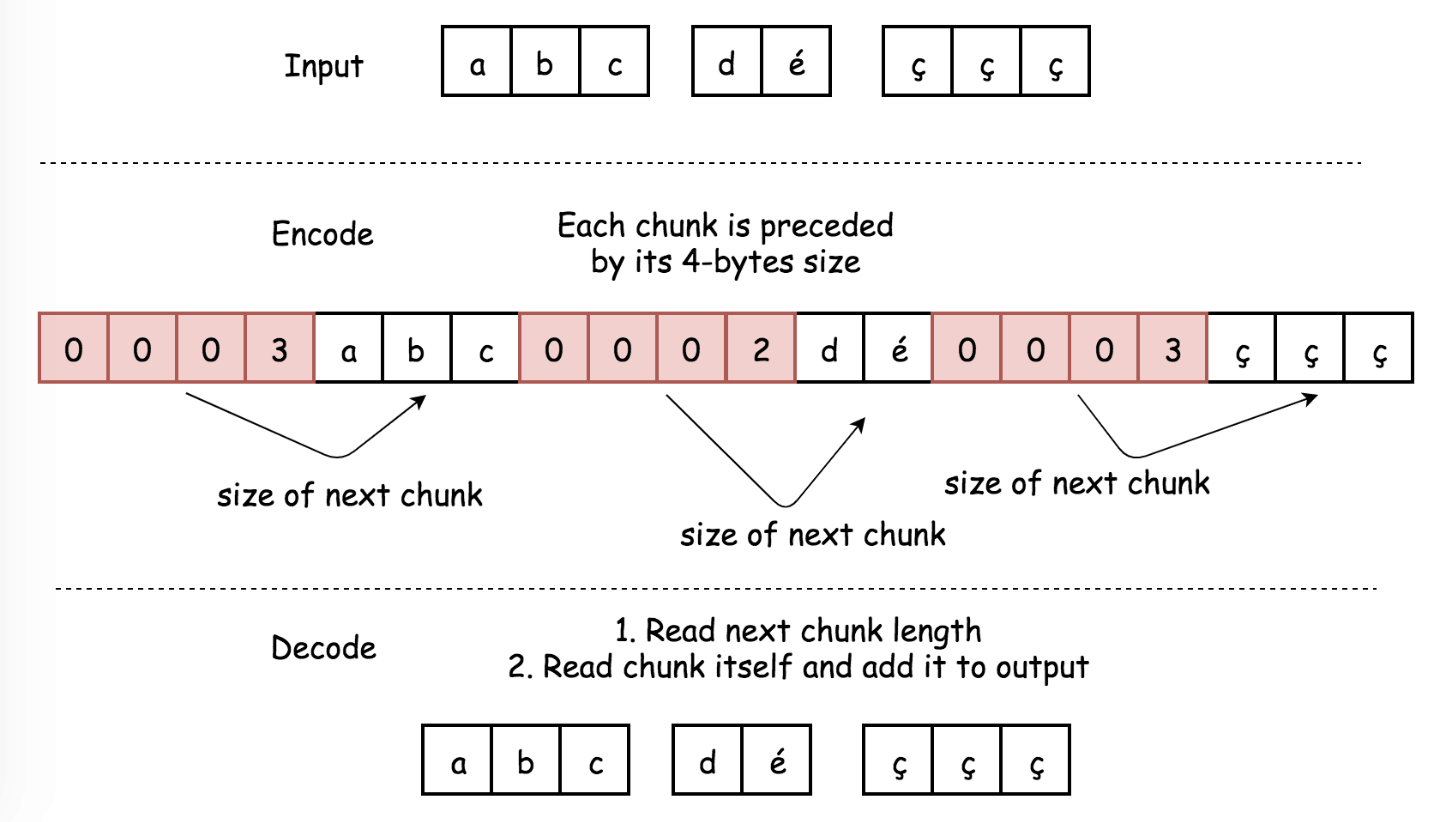# 271. Encode and Decode Strings

Reference: LeetCode
Difficulty: Medium

## Problem

Design an algorithm to encode a list of strings to a string. The encoded string is then sent over the network and is decoded back to the original list of strings.

Note:

• The string may contain any possible characters out of 256 valid ascii characters. Your algorithm should be generalized enough to work on any possible characters.
• Do not use class member/global/static variables to store states. Your encode and decode algorithms should be stateless.
• Do not rely on any library method such as eval or serialize methods. You should implement your own encode/decode algorithm.

## Analysis

In Java, The limit parameter controls the number of times the pattern is applied and therefore affects the length of the resulting array. We have 3 possible values for this limit:

1. If the limit is n > 0 then the pattern will be applied at most n - 1 times, which means the number of pieces will be no greater than n, and the array’s last entry will contain all input beyond the last matched delimiter ("" will be kept). For example, "..".split(".", 2) -> ["", "."].
2. If the limit is n < 0 then the pattern will be applied as many times as possible and the array can have any length. Besides, it will keep empty strings. For example, "..".split(".", -1) -> ["", "", ""].
3. If the limit is n == 0 (default) then the pattern will be applied as many times as possible and the array can have any length. Besides, it will delete empty strings. For example, "..".split(".", 0) -> [].

Methods:

Reference: Solution Post

1. Non-ASCII Delimiter
• Since the string may contain every possible 256 valid ASCII characters, we should choose an appropriate delimiter. For example, we can use non-ASCII unichar character like ā (Character.toString((char) 257)).
• It’s convenient to use two different non-ASCII characters, to distinguish between situations of empty array and of array of empty strings. So we use one more unichar character Character.toString((char) 258).

Time: $O(N)$
Space: $O(1)$ for encode; $O(N)$ for decode.

1. Chunked Transfer Encoding
• This approach is based on the encoding used in HTTP v1.1. It doesn’t depend on the set of input characters, and hence is more versatile and effective.
• Data stream is divided into chunks. Each chunk is preceded by its size in bytes.
• Encode:• For each chunk compute its length, and convert that length into 4-byte string.
• Append to encoded string:
• 4-byte string with information about chunk size in bytes.
• Chunk itself.
• Return encoded string.
• Decode:• Iterate over the encoded string with a pointer i:
• Read 4 bytes s[i: i + 4]. This chunk should be converted into integer length.
• Move the pointer by 4 bytes i += 4.
• Append to the decoded array string s[i: i + length].
• Move the pointer by length bytes i += length.
• Return decoded array of strings.

Time: $O(N)$
Space: $O(1)$ for encode to keep the output; $O(N)$ for decode to keep the output.

CommentJunhao Wang
a software engineering cat